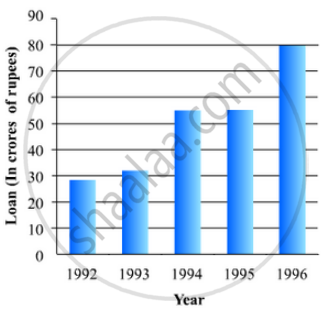# The Following Data Gives the Amount of Loans (In Crores of Rupees) Disbursed by a Bank During Some Years: - Mathematics

The following data gives the amount of loans (in crores of rupees) disbursed by a bank during some years:

 Year 1992 1993 1994 1995 1996 Loan(in crores of rupees) 28 33 55 55 80

(i) Represent the above data with the help of a bar graph.
(ii) With the help of the bar graph, indicate the year in which amount of loan is not increased over that of the preceding year.

#### Solution

To represent the given data by a vertical bar graph, we first draw horizontal and vertical axes. Let us consider that the horizontal and vertical axes represent the years and the amount of loan in Crores of rupees respectively. We have to draw 5 bars of different lengths given in the table.

At first we mark 5 points in the horizontal axis at equal distances and erect rectangles of the same width at these points. The heights of the rectangles are proportional to the amount of loan disbursed by the bank.

(1) The vertical bar graph of the given data is following:(2) It is seen from the bar graph that the heights of the bars in the years 1994 and 1995 are same.

Hence, the amount of loan is not increased in the year 1995 over the preceding year 1994.

Concept: Graphical Representation of Data
Is there an error in this question or solution?

#### APPEARS IN

RD Sharma Mathematics for Class 9
Chapter 23 Graphical Representation of Statistical Data
Exercise 23.2 | Q 7 | Page 26

Share